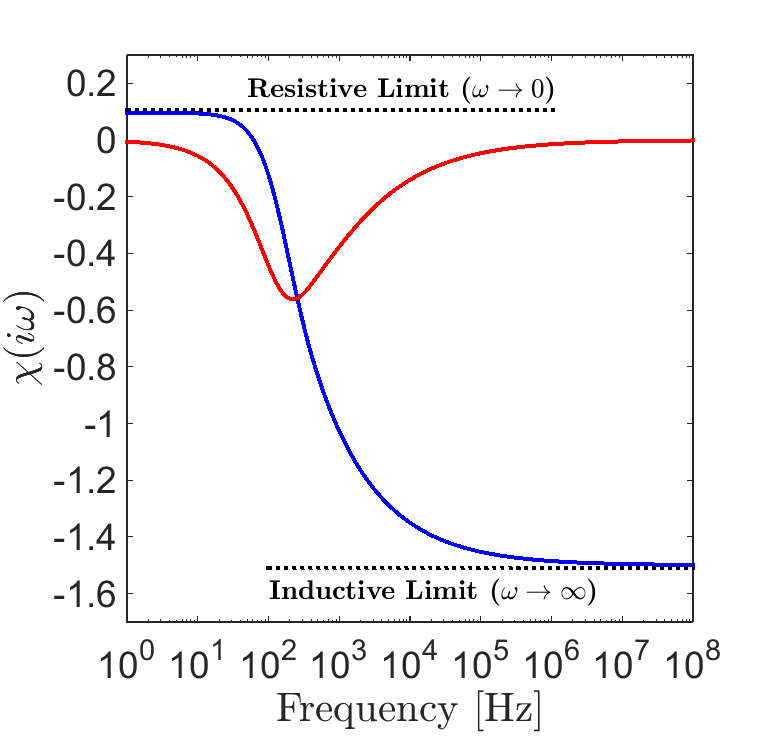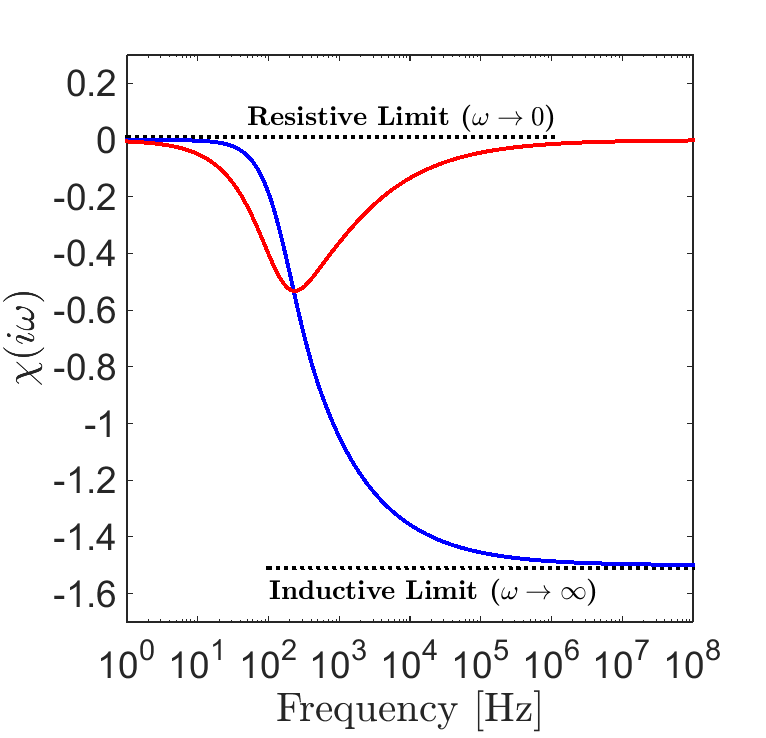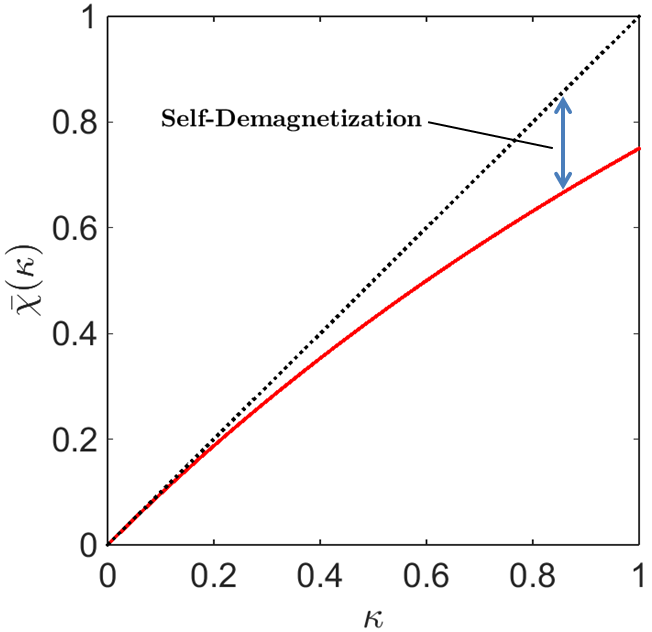# Excitation Factor for Special Cases

Purpose

The characteristic dipole response from a conductive and magnetically permeable sphere is defined by its excitation factor. Here, we present analytic expressions and discuss the excitation factors for several specific cases, including: a conductive and magnetically permeable sphere, a purely conductive sphere, and the zero-frequency response from a highly permeable sphere.

## Conductive and Magnetically Permeable Sphere

According to Wait ([Wai51]), the excitation factor for a conductive and magnetically permeable sphere is given by:

(386)$\chi (i \omega) = \frac{3}{2} \Bigg [ \! \frac{2\mu \big [ tanh(\alpha) - \alpha \big ] + \mu_0 \big [\alpha^2 \, tanh(\alpha) - \alpha + tanh(\alpha) \big ] }{\mu \big [ tanh(\alpha) - \alpha \big ] - \mu_0 [ \alpha^2 \, tanh(\alpha) - \alpha + tanh(\alpha) \big ] } \! \Bigg ]$

where

$\alpha = \Big [ i\omega\mu\sigma \Big ]^{1/2}R$Fig. 123 Excitation factor for a conductive and magnetically permeable sphere in free-space with $$R$$ = 25 m, $$\sigma$$ = 10 S/m, and $$\mu$$ = 1.1 $$\mu_0$$.

The excitation factor for a sphere with $$R$$ = 25 m, $$\sigma$$ = 10 S/m and $$\mu$$ = 1.1 $$\mu_0$$, can be seen in Fig. 123. At low frequencies, $$\chi (i\omega)$$ is positive, implying the excitation of the sphere is parallel to the inducing field. Because the EM induction is negligible at sufficiently low frequencies, this case represents a purely magnetic response by the sphere. At high frequencies, $$\chi(i\omega)$$ is negative. Therefore, inductive excitation of the sphere will oppose the inducing field. The inductive and resistive limits of the excitation factor can be obtained by taking the limits of Eq. (386):

(387)$\begin{split}\chi (i\omega) = \begin{cases} \dfrac{3 \big ( \mu - \mu_0 \big )}{ \big ( \mu + 2 \mu_0 \big ) } & \textrm{ as } \omega \rightarrow 0 \\ \\ - \dfrac{3}{2} & \textrm{ as } \omega \rightarrow \infty \end{cases}\end{split}$

Thus regardless of the sphere’s physical properties, the inductive limit of the sphere’s excitation factor is the same, whereas at the resistive limit, it depends on the sphere’s magnetic properties.

## Purely Conductive Sphere

For a purely conductive object (i.e. $$\mu = \mu_0$$), Eq. (386) can simplified to the following:

(388)$\chi (\omega) = - \; \frac{3}{2} \Bigg [ 1 + \frac{3}{\alpha^2} - \frac{3 \, \textrm{coth}(\alpha)}{\alpha} \Bigg ]$Fig. 124 Excitation factor for a conductive sphere in free-space with $$R$$ = 25 m, $$\sigma$$ = 10 S/m, and $$\mu$$ = $$\mu_0$$.

The excitation factor for a sphere with $$R$$ = 25 m, $$\sigma$$ = 10 S/m and $$\mu$$ = $$\mu_0$$, can be seen in Fig. 124. Because the sphere is non-permeable, there is no magnetic response at low frequencies. Thus we expect $$\chi (i\omega) \rightarrow 0$$ as $$\omega \rightarrow 0$$. At higher frequencies however, the sphere’s inductive response is approximately equal to that of the previous case. This can be shown by taking the inductive and resistive limits of Eq. (388):

(389)$\begin{split}\chi (i\omega) = \begin{cases} \; \; 0 & \textrm{ as } \omega \rightarrow 0 \\ \\ -\dfrac{3}{2} & \textrm{ as } \omega \rightarrow \infty \end{cases}\end{split}$

Therefore, a purely conductive sphere will only experience excitations which oppose the inducing field.

## Low-Frequency Limit for Highly Permeable Spheres

The excitation factor for a highly permeable sphere at low frequency can be obtained by examining the resistive limit of Eq. (386). Where $$\kappa$$ is the magnetic susceptibility (link) of the sphere, and $$\mu =\mu_0 \big [ 1 + \kappa \big ]$$:

(390)$\lim_{\omega \rightarrow 0} \; \chi (i\omega) = \dfrac{3 \big ( \mu - \mu_0 \big )}{ \big ( \mu + 2 \mu_0 \big ) } = \dfrac{3 \kappa }{3 + \kappa} = \bar \chi (\kappa)$Fig. 125 Zero-frequency excitation facter at $$\omega$$ = 0 for increasing magnetic susceptibilities (red), compared to a linear trend with respect to $$\kappa$$ (black).

$$\bar \chi (\kappa)$$ represents the zero-frequency excitation factor for a permeable sphere, and depends on the sphere’s magnetic susceptibility. The zero-frequency excitation factor $$\bar \chi (\kappa)$$, as a function of $$\kappa$$ is plotted in Fig. 125.

For small magnetic susceptibilities ($$\kappa < 0.1$$), the relationship between $$\kappa$$ and the excitation factor is approximately linear. For large values however, the effects of self-demagnetization (link) within the sphere will result in a proportionally weaker induced dipole moment. The effects of self-demagnetization for the sphere are given by:

(391)$\textrm{S. D.} = \kappa - \bar \chi (\kappa) = \frac{\kappa^2}{3 + \kappa}$

The largest possible magnetic response from a sphere can be obtained by taking the limit of Eq. (387) as $$\kappa \rightarrow \infty$$:

(392)$\lim_{\kappa \rightarrow \infty} \, \bar \chi (\kappa) = 3.$